Home > electronic components > capacitors > capacitors in series combination

# Capacitors in Series Combination

When one electrode of capacitor is connected to other electrode and the remaining electrodes of the capacitor are free to connect in the circuit that combination of capacitors is called as series connection of the capacitors. Since in series connection the current flowing through the circuit is same in all the components, the charging current flowing through the capacitors is same for all the capacitors. Therefore, in series combination of the capacitors current flowing through the capacitors is,From the above equation it can be seen that, the capacitor connected in circuit stores equal amount of electrical charge. This indicates that, although the capacitance of each capacitor is different the charge stored on the plates of the capacitor is equal in all the capacitors. Thus the charge stored on the plates of the capacitor is given by following equation,Figure below shows the series combination of four capacitors. The capacitors are named as C1, C2, C3 and C4 In this circuit the right hand side electrode of the first capacitor, C1 is connected to the left hand side electrode of the second capacitor, C2 .The right hand side electrode of C2 is connected to the left hand side electrode of the third capacitor, C3. The right hand side electrode of C3 is connected to the left hand side electrode of the fourth capacitor, C4. Further, the left hand side electrode of the first capacitor, C1 is connected to the positive terminal of DC battery and the right hand side electrode of the fourth capacitor, C4 is connected to the negative terminal of DC battery.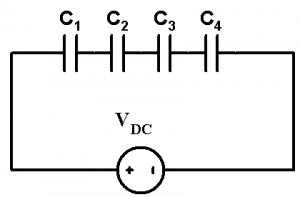In the above figure,
For Capacitor C1, the voltage across the capacitor is V1, charge stored in the capacitor is Q1 and current through the capacitor is I1.
For Capacitor C2, the voltage across the capacitor is V2, charge stored in the capacitor is Q2 and current through the capacitor is I2.
For Capacitor C3, the voltage across the capacitor is V3, charge stored in the capacitor is Q3 and current through the capacitor is I3.
For Capacitor C4, the voltage across the capacitor is V4, charge stored in the capacitor is Q4 and current through the capacitor is I4.
As we know that, in series connection the current flowing through the circuit is same in all the components, the charging current flowing through the capacitors is same for all the capacitors.
Therefore,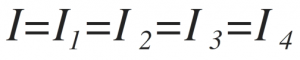From the above equation it can be seen that, the capacitor connected in circuit stores equal amount of electrical charge.
Therefore,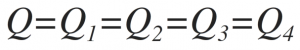Now, the voltage across the capacitors is given by,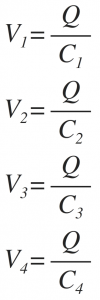From above equations, it can be seen that the voltage drop across the capacitors is different. As we know that, in series combination the current flowing through the circuit is same and the voltage drop across the series components is different. The total voltage applied by the battery is given by,As we know that V = Q/C, by using this equation we get,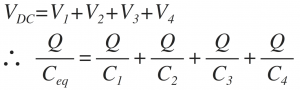Divide throughout by Q,
We get,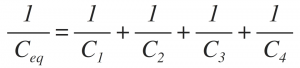The capacitor Ceq is the equivalent capacitance of the series combination of the capacitances. Instead of four if n number of capacitances are connected in series then the equivalent capacitance is given by,Now when two capacitors C1 and C2 are connected in series then the equivalent capacitance of this series combination is given by,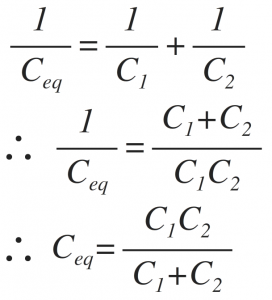From the above equation it can be seen that, in series combination of capacitors the equivalent capacitance of capacitors is lower than the value of the least value of the capacitor in series combination.

s